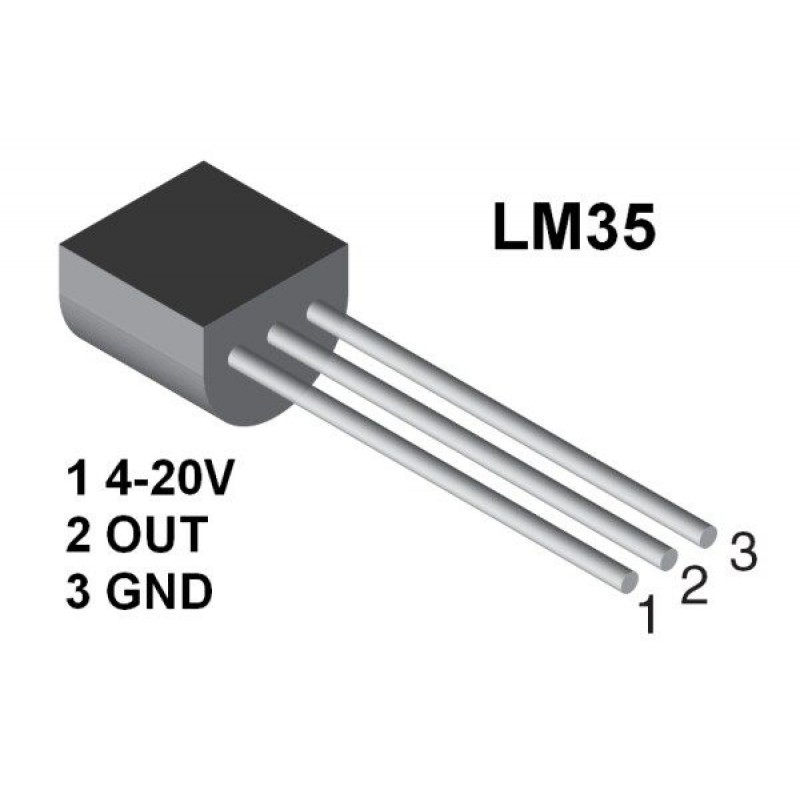# Temperature sensor LM35 and Arduino

LM35 is an analog type temperature sensor, whose output voltage varies linearly with change in temperature; LM35 is a three terminal sensor. Here we will see how to interface temperature sensor and arduino to display output on the LCD.

It is capable of measuring temperature from –55 degree Celsius to +150 degree Celsius. The output voltage of the LM35 increases 10mV per degree Celsius rise in temperature. LM35 operates with a 5V supply. The pin diagram of LM35 is shown in the figure below.Note: LM35 is available in the market in 3 series variations – LM35A, LM35C and LM35D series. The main difference between these 3 versions of LM35 IC is in their range of temperature measurements.

1. The LM35D series is designed to measure from 0 degree Celsius to 100 degree Celsius, whereas
2. LM35A series is designed to measure a wider range of –55 degree Celsius to 155 degree Celsius.
3. The LM35C series is designed to measure from –40 degree Celsius to 110 degree Celsius.

We are using the LM35Dz sensor which is category 1

### WORKING TEMPERATURE SENSOR AND ARDUINO

Arduino Uno and LM35 can be connected as shown in circuit diagram.LM35 is an analog sensor, so the output of LM35 is an analog signal. Microcontrollers are not capable of reading analog signals as their input directly. We need to convert this analog signal to digital before we can feed it to a microcontroller’s input. For that, we can use an ADC chip (Analog to Digital Converter).

If we are using a microcontroller like 8051 etc., we need to use an external ADC to convert analog output of LM35 to digital. Then, we feed the output of ADC (converted digital value) to input of 8051. But modern day development boards like Arduino and most modern day microcontrollers come with inbuilt ADC.

Our Arduino Uno has an in-built 10 bit ADC (6 channel). We can make use of this inbuilt ADC of arduino to convert the analog output of LM35 (or other device/sensor) to digital output.As Arduino Uno has a 6 channel inbuilt ADC, there are 6 analog input pins numbered from A0 to A5. You can connect analog out of LM35 to any of these analog input pins of an arduino but we are using A0 for now.

### CONNECTION TABLE

 Arduino pin LCD POT GND 1 – VSS + 5V 2 – VDD/VCC 3 – VEE POT 2 4 – RS (Reset) GND 5 – RW (Read/Write) 3 6 – E (Enable) – 7 – D0 – 8 – D1 – 9 – D2 – 10 – D3 4 11 – D4 5 12 – D5 6 13 – D6 7 14 – D7 + 5V 15 – Anode (+) GND 16 – Cathode (–)

 Arduino pin LM35 temperature Sensor +5V VCC GND GND A0 VOUT

### REQUIRED HARDWARE

 S.No. Item Quantity 1 LM35 temperature sensor 1 2 POT 10K 1 3 LCD 1 4 Arduino Board 1 5 Breadboard 1 6 Battery 5V / 9V 1 7 Male to Female jumper 14 8 Male to Male 3

## TEMPERATURE SENSOR ARDUINO CODE

In this Arduino programming code for temperature sensor LM35 will we print ADC value read by Arduino and then we will convert it into millivolt and print it as well.

Other than these we will also display degree symbol on 16×2 LCD using char print at value 223. To print more characters using the same concept follow this link print ASCII characters on LCD 16×2 using Arduino.

You can design your custom character on LCD 16×2 to do that you should follow this link display Hindi or custom character on LCD 16×2 using Arduino.

```// Tutorial: https://pijaeducation.com/arduino/sensor/temperature-sensor-and-arduino/

#include<LiquidCrystal.h>
LiquidCrystal lcd(2, 3, 4, 5, 6, 7); //(RS, E, D4, D5, D6, D7)
int ar = 0, v = 0, t = 0;
char y = 223;
void setup() {
lcd.begin(16, 2);
}

void loop() {
delay(100);
// how to convert analog to digital see here: https://pijaeducation.com/adc-in-arduino/
v = ar * 4.887;
t = v / 10;

lcd.setCursor(0, 0);
lcd.print("AR:");
lcd.println(ar);

lcd.setCursor(8, 0);
lcd.print("mV:");
lcd.println(v);

lcd.setCursor(0, 1);
lcd.print("Temperature:");
lcd.print(t);
lcd.print(y);

delay(1000);
lcd.clear();
}
```

### OUTPUT

As temperature increases or decreases it will be shown on the LCD screen. You can use this project as a Room temperature Monitoring system.SOIL SENSOR WITH ARDUINO IN ANALOG MODE

Subscribe
Notify of1 Comment
Inline Feedbacks